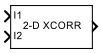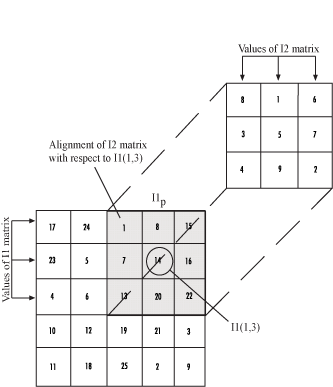# 2-D Correlation

Compute 2-D correlation of two input matrices

• Library:
• Computer Vision Toolbox / Statistics

•## Description

The 2-D Correlation block computes the two-dimensional cross-correlation between two input matrices.

## Ports

### Input

expand all

First input matrix, specified as a M-by-N matrix.

Data Types: `single` | `double` | `int8` | `int16` | `int32` | `int64` | `uint8` | `uint16` | `uint32` | `uint64` | `fixed point`
Complex Number Support: Yes

Second input matrix, specified as a P-by-Q matrix.

Data Types: `single` | `double` | `int8` | `int16` | `int32` | `int64` | `uint8` | `uint16` | `uint32` | `uint64` | `fixed point`
Complex Number Support: Yes

### Output

expand all

Calculated cross-correlation, returned as a scalar, vector, or matrix. The size of the cross-correlation output depends on the Output size parameter.

Data Types: `single` | `double` | `int8` | `int16` | `int32` | `int64` | `uint8` | `uint16` | `uint32` | `uint64` | `fixed point`
Complex Number Support: Yes

## Parameters

expand all

Main Tab

Size of the cross-correlation output.

• If you choose `Full`, the output has the dimensions (M+P-1)-by-(N+Q-1).

• If you choose `Same as input port I1`, the output has the same dimensions as the input at port I1. The block returns the central part of cross-correlation matrix, which is the same size as the input at port I1.

• If you choose `Valid`, the output has the dimensions (M-P+1)-by-(N-Q+1). The block returns only parts of the cross-correlation matrix that are computed without zero-padded edges.

Select this parameter, if the data types of the inputs are floating-point. The values of the cross-correlation output are normalized to [0, 1].

Data Types Tab

For details on the fixed-point block parameters, see Specify Fixed-Point Attributes for Blocks.

Select this parameter to prevent the fixed-point tools from overriding the data types you specify on this block. For more information, see Lock the Output Data Type Setting (Fixed-Point Designer).

## Block Characteristics

 Data Types `double` | `fixed point` | `integer` | `single` Multidimensional Signals `no` Variable-Size Signals `yes`

## Algorithms

Given two input matrices, I1 and I2, that are size M-by-N and P-by-Q, the 2-D cross-correlation value at the point `(k,l)` is given by

`$C\left(k,l\right)=\text{\hspace{0.17em}}\sum _{m=0}^{M-1}\sum _{n=0}^{N-1}I1\left(m,n\right)\overline{I2}\left(m+k,n+l\right).$`

The normalized cross-correlation value at the point `(k,l)` is calculated as

`$\begin{array}{l}{C}_{N}\left(k,l\right)=\text{\hspace{0.17em}}\frac{\sum _{m=0}^{M-1}\sum _{n=0}^{N-1}I1\left(m,n\right)\overline{I2}\left(m+k,n+l\right)}{\sqrt{\sum _{m=0}^{M-1}\sum _{n=0}^{N-1}I1{\left(m,n\right)}^{2}}\sqrt{\sum _{m=0}^{M-1}\sum _{n=0}^{N-1}\overline{I2}{\left(m+k,n+l\right)}^{2}}}\text{\hspace{0.17em}}\text{\hspace{0.17em}},\text{\hspace{0.17em}}\\ \text{where,}\text{\hspace{0.17em}}\\ 0\le k`

Suppose I1 and I2 are matrices with dimensions (4,3) and (2,2). The following figure shows how the block computes cross-correlation value for the point `I1(1,3)`, which refers to the second column and fourth row in zero-based indexing.The cross-correlation value for the point `I1(1,3)` is computed using these steps:

1. Slide the center element of I2 so that it lies on top of the (0,2) element of I1.

2. Multiply each weight in I2 by the element of I1 underneath.

3. Sum the individual products from step 2.

The cross-correlation value for the point `I1(1,3)` is $1\cdot 8+8\cdot 1+15\cdot 6+7\cdot 3+14\cdot 5+16\cdot 7+13\cdot 4+20\cdot 9+22\cdot 2=585$.

The normalized cross-correlation value for the point `I1(1,3)` is

`$\frac{585}{\sqrt{\sum I{1}_{p}^{2}}\sqrt{\sum I{2}^{2}}}\text{\hspace{0.17em}}=\text{\hspace{0.17em}}0.8070.$`